# Example calculation of agreement and disagreement between posterior distributions¶

Marco Raveri (mraveri@sas.upenn.edu)

This notebook shows an end to end calculation of the agreement and disagreement between two experiments with different methods, in an idealized case and in a realistic case involving two cosmological experiments.

In :
# Initial setup:
%matplotlib inline
%config InlineBackend.figure_format = 'retina'
# import libraries:
import sys, os
sys.path.insert(0,os.path.realpath(os.path.join(os.getcwd(),'../..')))
from getdist import plots, MCSamples
from getdist.gaussian_mixtures import GaussianND
import getdist
import scipy
import matplotlib.pyplot as plt
import IPython
import numpy as np
# import tension tools utilities:
from tensiometer import utilities


## Toy example:¶

For a toy example we consider two 2D Gaussian distributions. One has correlation between the two parameters while the other does not. The two Gaussian distributions are displaced a little to result in a mild tension.

In :
# Create the two toy chains and the joint chain:
ndim = 2 # number of dimensions
nsamp = 10000 # number of samples
nchains = 8 # number of chains
# helper function to compute the likelihood:
def helper_gaussian_log_like( samps, mean, invcov ):
_diff = samps-mean
return (_diff.dot(invcov)*_diff).sum(axis=1)
# first data set:
mean_1 = np.array([0., 0.])
cov_1 = np.array([[1, -0.9], [-0.9, 1]])
samps_1 = np.random.multivariate_normal(mean_1, cov_1, size=nchains*nsamp)
log_like_1 = helper_gaussian_log_like(samps_1, mean_1, np.linalg.inv(cov_1))
# second data set:
mean_2 = np.array([1., 1.])
cov_2 = np.array([[.09, 0.], [0., .09]])
samps_2 = np.random.multivariate_normal( mean_2, cov_2, size=nchains*nsamp)
log_like_2 = helper_gaussian_log_like(samps_2, mean_2, np.linalg.inv(cov_2))
# joint data set:
cov_12 = np.linalg.inv(np.linalg.inv(cov_1) + np.linalg.inv(cov_2))
mean_12 = np.dot(cov_12, np.dot(np.linalg.inv(cov_1), mean_1) + np.dot(np.linalg.inv(cov_2), mean_2))
samps_12 = np.random.multivariate_normal(mean_12, cov_12, size=nchains*nsamp)
log_like_12 = helper_gaussian_log_like(samps_12, mean_1, np.linalg.inv(cov_1)) \
+ helper_gaussian_log_like(samps_12, mean_2, np.linalg.inv(cov_2))
# initialize the parameter names:
names = ["p%s"%i for i in range(ndim)]
labels = ["p_%s"%i for i in range(ndim)]
# initialize the GetDist chains:
chain_1 = MCSamples(samples=samps_1, loglikes=log_like_1, names=names, labels=labels, label='first')
chain_2 = MCSamples(samples=samps_2, loglikes=log_like_2, names=names, labels=labels, label='second')
chain_12 = MCSamples(samples=samps_12, loglikes=log_like_12, names=names, labels=labels, label='joint')
# separate the chains so that we can have rough convergence estimates:
for chain in [chain_1, chain_2, chain_12]:
chain.chain_offsets = [i*nsamp for i in range(nchains+1)]


Now we plot the distributions to make sure we are getting a good example:

In :
g = plots.get_subplot_plotter()
g.triangle_plot([chain_1, chain_2, chain_12], filled=True)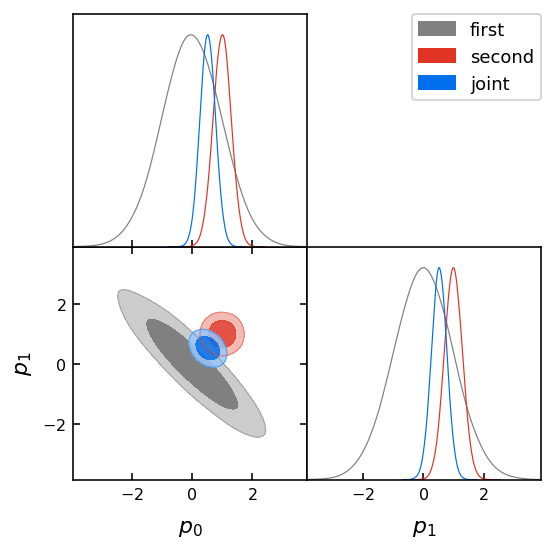Clearly if we were to look only at the marginalized posteriors of the two distributions we would probably not guess that the two chains are in tension with each other. We next proceed to the calculation of the statistical significance of this tension.

### Parameter shift exact (MCMC) estimates:¶

We first compute the distribution of parameter shifts and get its compatibility with zero. Since the two input distributions are Gaussian we can compare the results with the exact calculation.

For an indepth on the methods used for this calculation we refer to the example notebook that focuses on this type of calculation.

In :
# import the module:
from tensiometer import mcmc_tension
# set single thread for running on small machines:
# create the distribution of the parameter differences:
diff_chain = mcmc_tension.parameter_diff_chain( chain_1, chain_2, boost=1 )
diff_chain.name_tag = 'MCMC difference'
# create the analytic difference distribution to check:
diff_mean  = mean_1 -mean_2
diff_cov   = cov_1 + cov_2
diff_samps = np.random.multivariate_normal( diff_mean, diff_cov, size=nsamp)
diff_chain_analytic = MCSamples( samples = diff_samps,
names   = ["delta_p%s"%i for i in range(ndim)],
labels  = ["\\Delta p_%s"%i for i in range(ndim)],
label   = 'analytic difference' )

In :
# plot the two distributions:
g = plots.get_subplot_plotter()
g.triangle_plot([diff_chain, diff_chain_analytic], filled=True, markers={'delta_p0':0,'delta_p1':0})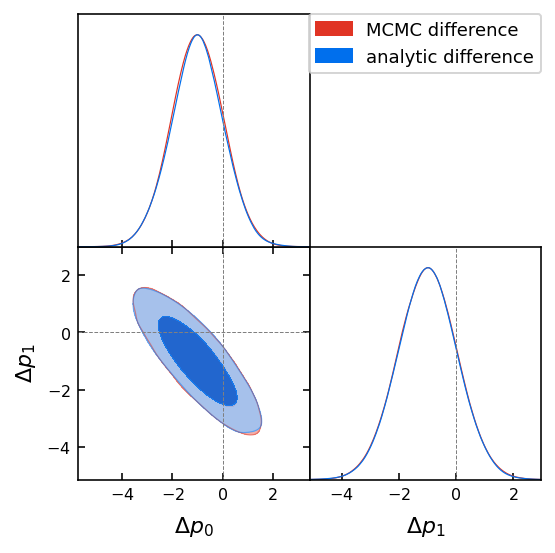As we can see the MCMC difference chain matches well the analytic difference chain. The difference chain is noisier than the input chains. The situation can be improved by boosting the number of samples in the parameter difference distribution at the expense of longer computation times.

We can now compute the statistical significance of this difference from zero. Since this example is in 2D we can use the FFT algorithm as follows.

In :
# compute the probability of shift:
shift_probability, shift_lower, shift_upper = mcmc_tension.kde_parameter_shift_2D_fft(diff_chain, feedback=0)

In :
# plot the contour for a sanity check:
g = plots.get_single_plotter()
g.settings.num_plot_contours = 3
g.plot_2d(diff_chain, 'delta_p0', 'delta_p1', filled=True );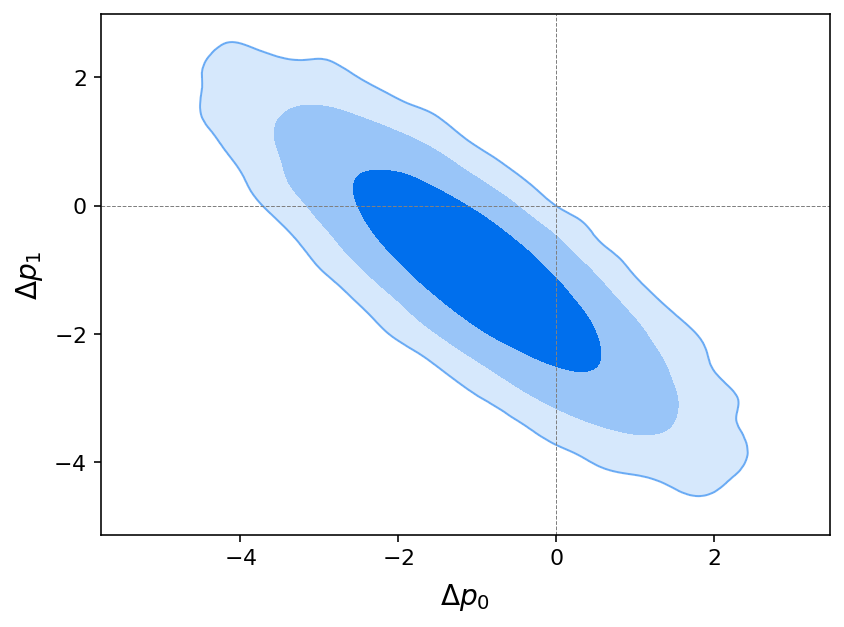This looks in very good agreement given that the difference chain is noisy and the plot smoothing is likely enlarging the contours. Since the input distributions are Gaussian we can check the results with the Gaussian case:

In :
# compute probability of a Gaussian shift:
chi2_probability = scipy.stats.chi2.cdf(np.dot(np.dot( diff_mean, utilities.QR_inverse(diff_cov) ), diff_mean ), 2 )
# print the results:
print(f'MCMC shift probability: {shift_probability:.5f} +{shift_upper-shift_probability:.5f} -{shift_probability-shift_lower:.5f}')
print(f'Gaussian probability: {chi2_probability:.5f}')
print(f'Difference: {np.abs(shift_probability-chi2_probability):.5f}')

MCMC shift probability: 0.99629 +0.00021 -0.00023
Gaussian probability: 0.99482
Difference: 0.00147


as we can see the two results agree very well but the error estimate from the samples is underestimating the difference with the exact result so the error estimate should not be trusted too much.

Finally we convert the results to the effective number of sigmas. This is the number of standard deviations that an event from a Gaussian distribution would have given our probability:

In :
# Then we convert the result to the effective number of sigmas:
print(f'MCMC shift nsigma: {utilities.from_confidence_to_sigma(shift_probability):.3f}')
print(f'Gaussian nsigma: {utilities.from_confidence_to_sigma(chi2_probability):.3f}')

MCMC shift nsigma: 2.902
Gaussian nsigma: 2.796


which agree fairly well, to a fraction of a sigma.

### Gaussian estimators:¶

We can proceed to compute the statistical significance of the difference in parameters with other estimators, as discussed in https://arxiv.org/abs/1806.04649 . These rely on the Gaussian approximation (though some of them are defined in a way that mitigates possible non-Gaussianities). We start with parameter shifts in standard form Q_DM and in update form Q_UDM:

In :
# import the module:
from tensiometer import gaussian_tension
# start calculations:
Q_DM, Q_DM_dofs = gaussian_tension.Q_DM( chain_1, chain_2 )
Q_UDM, Q_UDM_dofs =gaussian_tension.Q_UDM( chain_1, chain_12, lower_cutoff=1. )
Q_DM_P = scipy.stats.chi2.cdf(Q_DM, Q_DM_dofs)
Q_UDM_P = scipy.stats.chi2.cdf(Q_UDM, Q_UDM_dofs)
Q_DM_nsigma = utilities.from_confidence_to_sigma(Q_DM_P)
Q_UDM_nsigma = utilities.from_confidence_to_sigma(Q_UDM_P)
print(f'Q_DM = {Q_DM:.2f}, dofs = {Q_DM_dofs:2}, P = {Q_DM_P:.5f}, nsigma = {Q_DM_nsigma:.3f}')
print(f'Q_UDM = {Q_UDM:.2f}, dofs = {Q_UDM_dofs:2}, P = {Q_UDM_P:.5f}, nsigma = {Q_UDM_nsigma:.3f}')

Q_DM = 10.61, dofs =  2, P = 0.99504, nsigma = 2.809
Q_UDM = 10.63, dofs =  2, P = 0.99508, nsigma = 2.813


That we see match very well, to a fraction of a sigma.

We can now test the behavior of Q_UDM:

In :
# verify that Q_UDM is symmetric in the Gaussian case:
print('Symmetric Q_UDM = %.3f, dofs = %2i' % gaussian_tension.Q_UDM(chain_2, chain_12, lower_cutoff=1.))
# find the optimal cutoff by providing the second chain:
print('Q_UDM optimal cutoff = %.3f' % gaussian_tension.Q_UDM_get_cutoff(chain_1, chain_2, chain_12))
# get the KL spectrum:
print('Q_UDM KL spectrum:', gaussian_tension.Q_UDM_KL_components(chain_1, chain_12))

Symmetric Q_UDM = 10.761, dofs =  2
Q_UDM optimal cutoff = 1.000
Q_UDM KL spectrum: [22.14434443  2.10003097]


As we can see the estimator is symmetric, none of the parameters we are considering is prior limited and the optimal cutoff just leave all directions come through. We can proceed to compute the chi squared based version of these estimators. This consists in the statistics of Goodness of fit loss (Q_DMAP) which gives:

In :
Q_DMAP, Q_DMAP_dofs = gaussian_tension.Q_DMAP(chain_1, chain_2, chain_12, feedback=0 )
Q_DMAP_P = scipy.stats.chi2.cdf(Q_DMAP, Q_DMAP_dofs)
Q_DMAP_nsigma = utilities.from_confidence_to_sigma(Q_DMAP_P)
print(f'Q_DMAP = {Q_DMAP:.2f}, dofs = {Q_DMAP_dofs:2}, P = {Q_DMAP_P:.5f}, nsigma = {Q_DMAP_nsigma:.3f}')

Q_DMAP = 10.53, dofs = 2.0, P = 0.99482, nsigma = 2.796


Which is in very good agreement with the previous results, as expected.

## A realistic example, Planck 2018 and DES Y1:¶

We now turn to a more realistic example that involves a chains from two different (real) experiments. We use the LCDM parameter chains for the results of Planck 2018 (https://arxiv.org/abs/1807.06209) and the results for the Dark Energy Survey (DES) first year of data (https://arxiv.org/abs/1708.01530).

Notice that we have removed many parameters from the chains since they were irrelevant to the example. The chains are already fully polished. Burn in has been removed and the samples have been thinned.

Notice that we have run a prior only chain to ensure that the modeling of the prior is as faithful as possible. In particular, in standard cosmological analyses, we have priors on derived parameters that would give non-trivial shapes to the parameters that are being sampled (see Appendix F in https://arxiv.org/abs/1806.04649)

In :
# load the samples (remove no burn in since the example chains have already been cleaned):
chains_dir = './../../test_chains/'
# the Planck 2018 TTTEEE chain:
# the DES Y1 3x2 chain:
# the joint chain:
# the prior chain:


We first plot the chains to get some intuition of what is happening. We show only the parameters that have been run and that the two chains share. These are the only parameters that can contribute to a tension between the two experiments.

In :
shared_param_names = [name for name in chain_1.getParamNames().getRunningNames()
if name in chain_2.getParamNames().getRunningNames()]
g = plots.get_subplot_plotter()
g.triangle_plot([chain_1, chain_2, chain_12], params=shared_param_names, filled=True)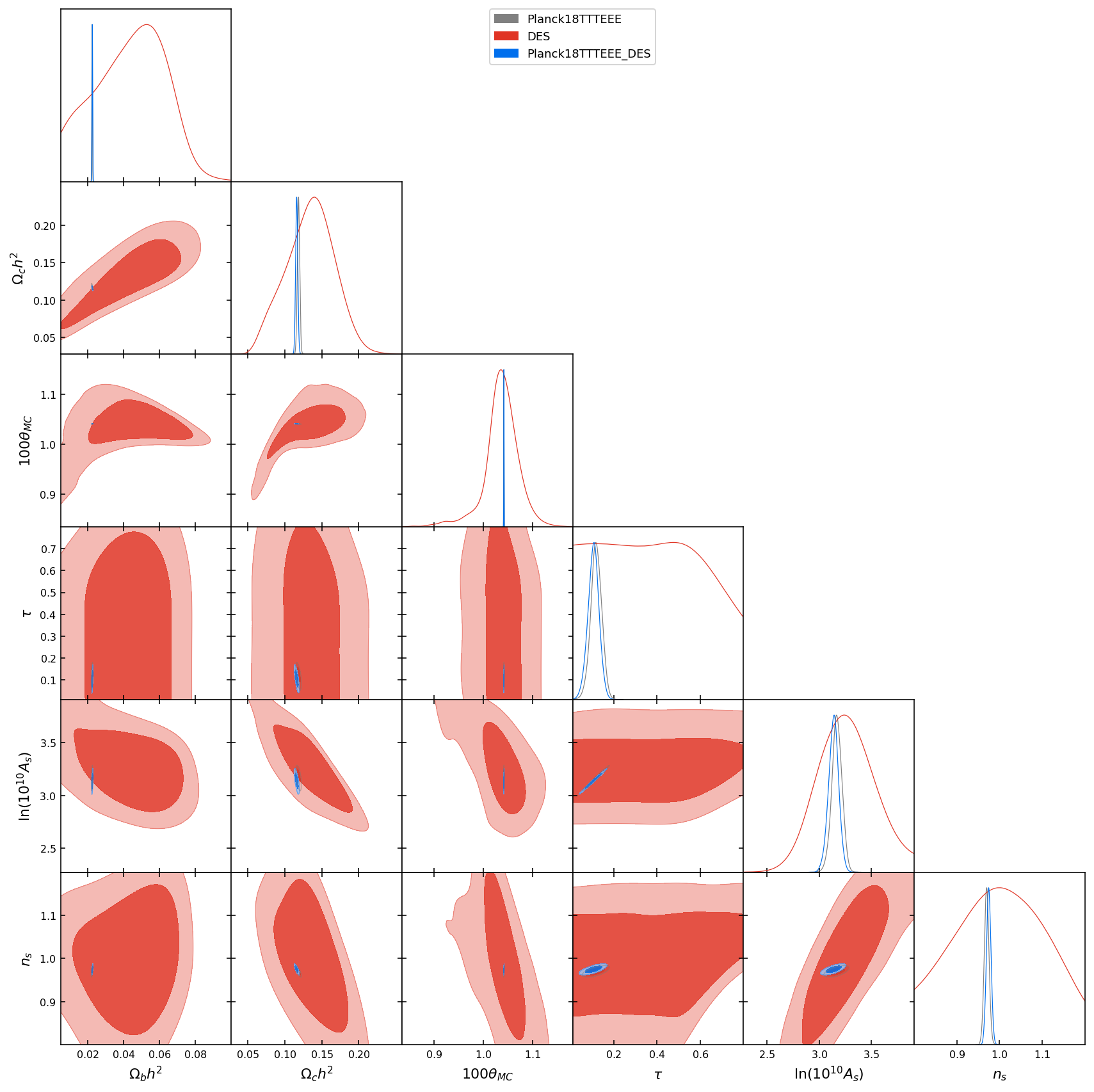Given this plot it is not obvious at all whether the two experiments agree or not.

Moreover, while Planck might still look Gaussian, DES looks like it has a very non-Gaussian posterior.

If we try another parameter combination:

In :
g = plots.get_subplot_plotter()
g.triangle_plot([chain_2, chain_1, chain_12], params=['sigma8','omegam'], filled=True)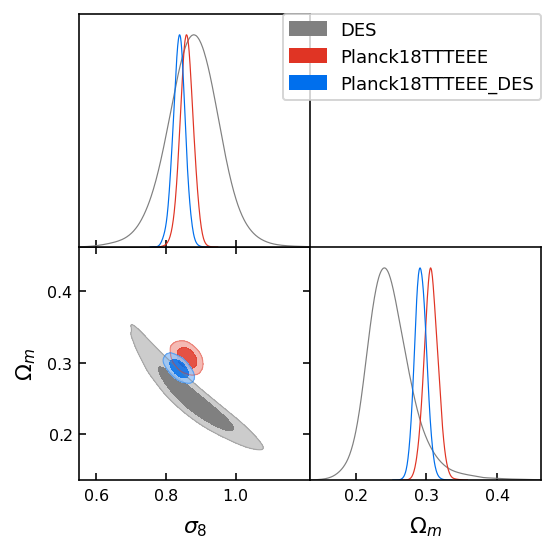The situation looks very different. We have selected the two parameters that the weakest data set (DES) best constrains and in this plane the two data sets do not look in agreement. They look fairly similar to our toy example! Coincidence?

The posteriors also look more Gaussian since we are selecting at the best constrained directions. This is a very common problem. When looking at some parameter that is partially informed by the prior the posterior might look non-Gaussian because of prior volume projections.

We can compute the number of parameters that our data sets are truly constraining over the prior.

In :
Neff_1 = gaussian_tension.get_Neff(chain_1, param_names=shared_param_names, prior_chain=prior_chain)
Neff_2 = gaussian_tension.get_Neff(chain_2, param_names=shared_param_names, prior_chain=prior_chain)
Neff_12 = gaussian_tension.get_Neff(chain_12, param_names=shared_param_names, prior_chain=prior_chain)
print(f'Neff(Planck) = {Neff_1:.2f}, Neff(DES) = {Neff_2:.2f}, Neff(joint) = {Neff_12:.2f}')

Neff(Planck) = 5.98, Neff(DES) = 3.31, Neff(joint) = 5.98


It is no surprise that Planck is constraining all six cosmological parameters while DES is constraining three. In addition to the two parameters, that we have shown in the plot above, DES is constraining the angular scale of the BAO peak, as we can see by directly feeding the parameters to use in the calculation:

In :
Neff_2 = gaussian_tension.get_Neff(chain_2, param_names=['sigma8','omegam','theta_BAO_DES'], prior_chain=prior_chain)
print(f'Neff(DES) = {Neff_2:.2f}')
# the extra 0.5 parameter is a very weak constraint on n_s.

Neff(DES) = 2.83


Since these are real data we can check the Goodness of their fit at maximum posterior.

In :
## in our case the DES data set has 457 data points and the Planck one has 2289
# if we were to normalize the likelihood we need to subtract the normalization factor to get the chi2.
Q_MAP_1 = gaussian_tension.Q_MAP(chain_1, num_data=2289, prior_chain=prior_chain)
Q_MAP_2 = gaussian_tension.Q_MAP(chain_2, num_data=457, prior_chain=prior_chain)
Q_MAP_12 = gaussian_tension.Q_MAP(chain_12, num_data=2289+457, prior_chain=prior_chain)
# get the probability:
Q_MAP_1_P = scipy.stats.chi2.cdf(*Q_MAP_1)
Q_MAP_2_P = scipy.stats.chi2.cdf(*Q_MAP_2)
Q_MAP_12_P = scipy.stats.chi2.cdf(*Q_MAP_12)
# print results:
print(f'Goodness of fit for Planck TTTEEE: Q_MAP = {Q_MAP_1:.3f} with dofs {Q_MAP_1:.3f}')
print(f'Q_MAP probability = {Q_MAP_1_P:.5f}, n_sigma = {utilities.from_confidence_to_sigma(Q_MAP_1_P):.3f}\n')
print(f'Goodness of fit for DES: Q_MAP = {Q_MAP_2:.3f} with dofs {Q_MAP_2:.3f}')
print(f'Q_MAP probability = {Q_MAP_2_P:.5f}, n_sigma = {utilities.from_confidence_to_sigma(Q_MAP_2_P):.3f}\n')
print(f'Goodness of fit for joint: Q_MAP = {Q_MAP_12:.3f} with dofs {Q_MAP_12:.3f}')
print(f'Q_MAP probability = {Q_MAP_12_P:.5f}, n_sigma = {utilities.from_confidence_to_sigma(Q_MAP_12_P):.3f}')

Goodness of fit for Planck TTTEEE: Q_MAP = 2337.482 with dofs 2283.021
Q_MAP probability = 0.79088, n_sigma = 1.256

Goodness of fit for DES: Q_MAP = 499.154 with dofs 453.685
Q_MAP probability = 0.93106, n_sigma = 1.819

Goodness of fit for joint: Q_MAP = 2850.685 with dofs 2740.021
Q_MAP probability = 0.93113, n_sigma = 1.819


This tells us that the maximum posterior is a fairly good fit to the data.

Then we check the reliability of the Gaussian approximation:

In :
# get the Gaussian approximation:
gaussian_1 = gaussian_tension.gaussian_approximation(chain_1)
gaussian_2 = gaussian_tension.gaussian_approximation(chain_2)
# plot for comparison:
g = plots.get_subplot_plotter()
g.triangle_plot([chain_1, chain_2, gaussian_1, gaussian_2], params=['sigma8','omegam','theta_BAO_DES'], filled=True)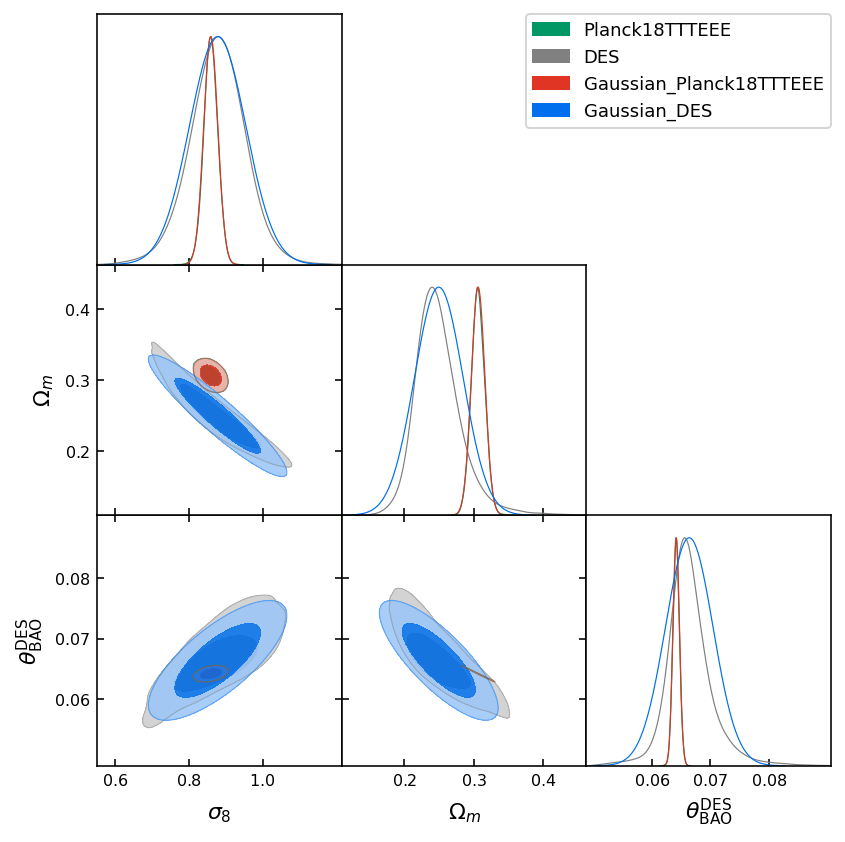This looks more Gaussian than the previous plot but still concerning because of clear non-Gaussianities.

### Parameter shift exact (MCMC) estimates:¶

We then proceed with the calculation of the distribution of parameter shifts that allows to take these non-Gaussianities fully into account. You can also look at the specific example notebook detailing the use of these types of estimators.

In :
# get the parameter difference chain:
diff_chain = mcmc_tension.parameter_diff_chain(chain_1, chain_2, boost=4)
# plot:
g = plots.get_subplot_plotter()
g.triangle_plot([diff_chain], params=['delta_sigma8','delta_omegam','delta_theta_BAO_DES'], filled=True, markers={'delta_sigma8':0,'delta_omegam':0,'delta_theta_BAO_DES':0})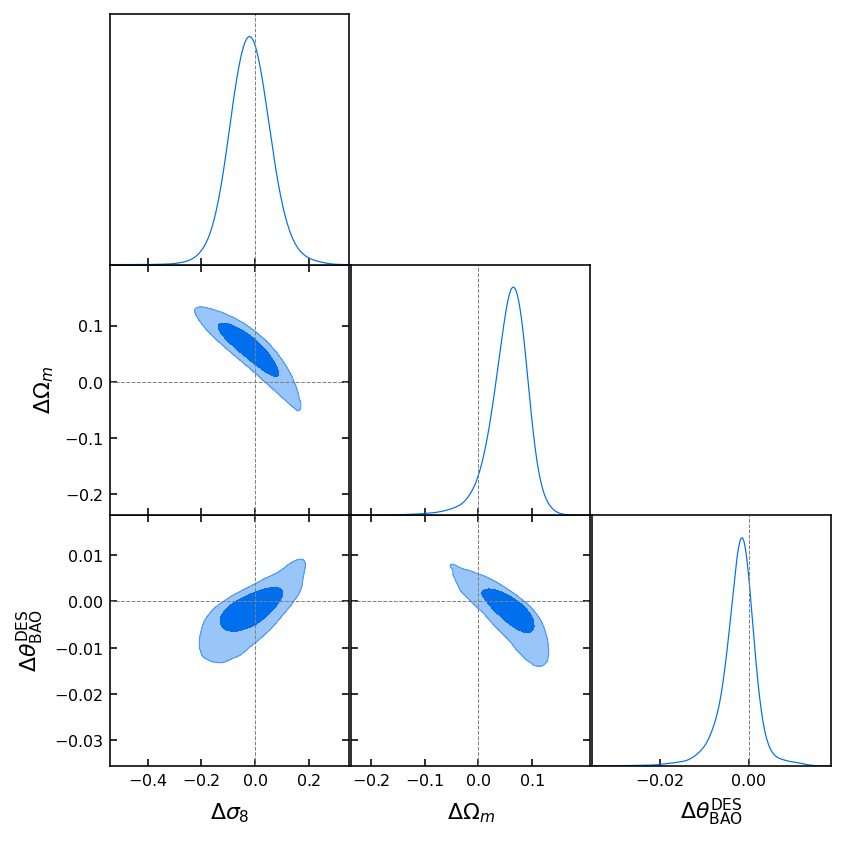We now compute the statistical significance of a shift considering the full parameter space (this will take some time):

In :
exact_shift_P_1, exact_shift_low_1, exact_shift_hi_1 = mcmc_tension.kde_parameter_shift(diff_chain, feedback=0)
print(f'Shift probability considering all parameters = {exact_shift_P_1:.5f} +{exact_shift_hi_1-exact_shift_P_1:.5f} -{exact_shift_P_1-exact_shift_low_1:.5f}')
# turn the result to effective number of sigmas:
print(f'    n_sigma = {utilities.from_confidence_to_sigma(exact_shift_P_1):.3f}')

Shift probability considering all parameters = 0.99659 +0.00005 -0.00005
n_sigma = 2.928

In :
exact_shift_P_2, exact_shift_low_2, exact_shift_hi_2 = mcmc_tension.kde_parameter_shift(diff_chain, feedback=0, param_names=['delta_sigma8', 'delta_omegam','delta_theta_BAO_DES'])
print(f'Shift probability considering selected parameters = {exact_shift_P_2:.5f} +{exact_shift_hi_2-exact_shift_P_2:.5f} -{exact_shift_P_2-exact_shift_low_2:.5f}')
# turn the result to effective number of sigmas:
print(f'    n_sigma = {utilities.from_confidence_to_sigma(exact_shift_P_2):.3f}')

Shift probability considering selected parameters = 0.99862 +0.00003 -0.00003
n_sigma = 3.198


As we can see the two results agreee to a fraction of a sigma since we have selected all the parameters that matter (i.e. are constrained by the data and not the prior). Adding n_s to the pool will account for the missing 0.2 sigma in the result. Notice, however, that the time it takes to work in the full parameter space is significantly higher.

We can make a sanity check plot looking at only the two most constrained parameters:

In :
g = plots.get_single_plotter()